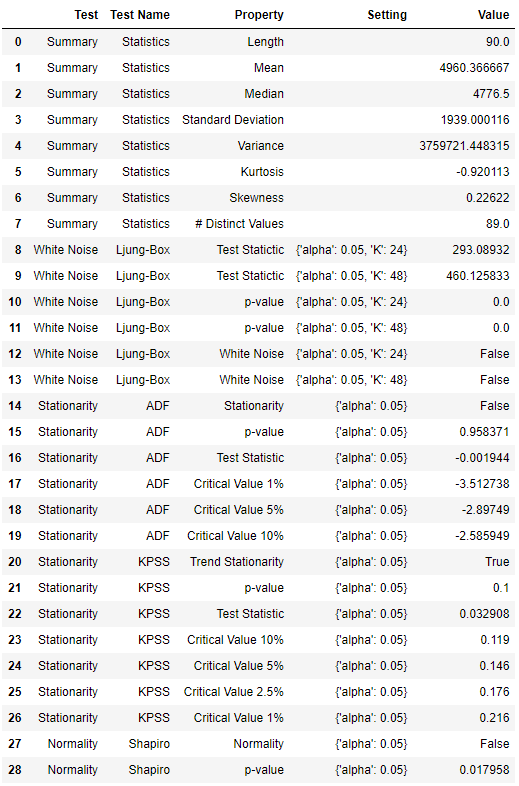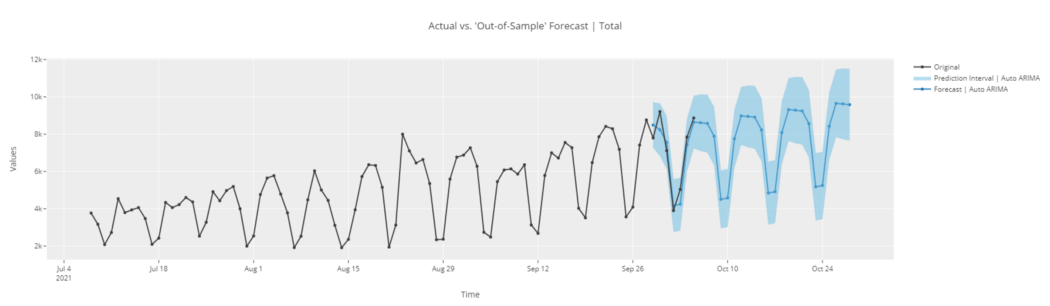﻿ pyCaret效率倍增开源低代码的python机器学习工具_python_脚本之家
python# pyCaret效率倍增开源低代码的python机器学习工具

PyCaret 是一个开源、低代码的 Python 机器学习库，可自动执行机器学习工作流。它是一种端到端的机器学习和模型管理工具，可以以指数方式加快实验周期并提高您的工作效率。欢迎收藏学习，喜欢点赞支持，文末提供技术交流群。

PyCaret 的设计和简单性受到数据科学家这一新兴角色的启发，可以执行以前需要更多技术专长的简单和中等复杂的分析任务。

## PyCaret 时间序列模块

PyCaret 的新时间序列模块现已提供测试版。 秉承 PyCaret 的简单性，它与现有的 API 保持一致，并带有很多功能。 统计测试、模型训练和选择(30 多种算法)、模型分析、自动超参数调优、实验记录、云部署等， 所有这一切只需要几行代码（就像 pycaret 的其他模块一样）。 如果您想尝试一下，请查看官方的快速入门笔记本。

```pip install pycaret-ts-alpha
```

PyCaret 的时间序列模块中的工作流程非常简单。 它从设置功能开始，您可以在其中定义预测范围 fh 和折叠次数。 您还可以将 fold_strategy 定义为扩展或滑动。

plot_model 函数可以在训练之前或之后使用。 在训练前使用时，它使用 plotly 界面收集了大量时间序列 EDA 图。 与模型一起使用时，plot_model 处理模型残差，并可用于访问模型拟合。

## 加载数据

```import pandas as pd
from pycaret.datasets import get_data
data['Date'] = pd.to_datetime(data['Date'])
data = data.groupby('Date').sum()
data = data.asfreq('D')
``````# plot the data
data.plot()
```## 初始化设置

```# with functional API
from pycaret.time_series import *
setup(data, fh = 7, fold = 3, session_id = 123)
# with new object-oriented API
from pycaret.internal.pycaret_experiment import TimeSeriesExperiment
exp = TimeSeriesExperiment()
exp.setup(data, fh = 7, fold = 3, session_id = 123)
```## 统计测试

```check_stats()
```## 探索性数据分析

```# functional API
plot_model(plot = 'ts')
# object-oriented API
exp.plot_model(plot = 'ts')
``````# cross-validation plot
plot_model(plot = 'cv')
``````# ACF plot
plot_model(plot = 'acf')
``````# Diagnostics plot
plot_model(plot = 'diagnostics')
``````# Decomposition plot
plot_model(plot = 'decomp_stl')
```## 模型训练和选择

```# functional API
best = compare_models()
# object-oriented API
best = exp.compare_models()
``````# create fbprophet model
prophet = create_model('prophet')
print(prophet)
```tune_model 也没有太大不同。

```tuned_prophet = tune_model(prophet)
print(tuned_prophet)
``````plot_model(best, plot = 'forecast')
``````# forecast in unknown future
plot_model(best, plot = 'forecast', data_kwargs = {'fh' : 30})
``````# in-sample plot
plot_model(best, plot = 'insample')
``````# residuals plot
plot_model(best, plot = 'residuals')
``````# diagnostics plot
plot_model(best, plot = 'diagnostics')
```## 保存模型

```# finalize model
final_best = finalize_model(best)
# generate predictions
predict_model(final_best, fh = 90)
``````# save the model
save_model(final_best, 'my_best_model')
```